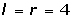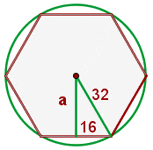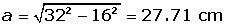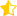A regular hexagon is a polygon with six equal sides and angles.

The triangles formed by joining the center with all the vertices, are equal in size and are equilateral.## Angles of the Hexagon

The sum of interior angles of a hexagon = (6 − 2) · 180° = 720°

The value of an interior angle of the regular hexagon is 720º/6 = 120º

The central angle of the regular hexagon measures: 360º : 6 = 60º

## Diagonals of the Hexagon

The number of diagonals = 6 · (6 − 3) : 2 = 9## Apothem of a Regular HexagonBy applying the Pythagorean theorem for one of the triangles, we obtain:## Perimeter of a Regular Hexagon

Perimeter = 6 · l## Area of a Regular HexagonCalculate the apothem, perimeter and area of a regular hexagon inscribed in a circle with a radius of 4 cm.P = 6 · 4 = 24 cmThe area of a square is 2,304 cm². Calculate the area of a regular hexagon that has the same perimeter as this square.Did you like the article?(2 votes, average: 4.50 out of 5)Loading...

Emma

I am passionate about travelling and currently live and work in Paris. I like to spend my time reading, gardening, running, learning languages and exploring new places.

Did you like
this resource?

Bravo!